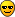# How might i fill Ntuple in 2D

Hi All,

``````TNtuple *ntuple = new TNtuple("basic","data from ascii","x:y:z");
ntuple->Fill(x,y,z) ``````

filling in 1D, OK.

But, How might i fill (not Draw!) Ntuple in 2D ?

For example, I tried this one,

Of course, I got an error.

Cheers,
Ersel

TNtuple *ntuple = new TNtuple(“basic”, “data from ascii”, “x:z”);
ntuple->Fill(x, z);

No, your code shows two branches in 1D ntuple, being separated from each other, doesn’t it ?

For example, for drawing ntuple, in 2D,

But i want to learn,in 2D, how to “fill” a branch of a ntuple !

Cheers,
Ersel

“x:y:z” is 3D (3 variables)
“x:z” is 2D (2 variables)ok i’ll try it. wait for it…Is there an option to fill first x ,then y according to ntuple->Fill(x,y) ?

For example, I tried to fill ntuple for implementing the option above ;

``````if (hv==0) { ntuple->Fill(x);}
if(hv==1) {ntuple->Fill(x,y);}``````

but here filled x twice!

…and also tried this one;

``````if (hv==0) { ntuple->Fill(x);}
if (hv==1) { ntuple->Fill(" ",y); }``````

of course, i got an errorI mean that, i look at option for TNtuple::Fill() Options, but i didn’t found anything about what i mention above, Is there an option to provide a facility ?

If you have two completely independent variables then you should create two TNtuple objects (so, a “1D” TNtuple for each variable).

Or you could create a single “2D” TNtuple:
TNtuple *ntuple = new TNtuple(“ntuple”, “HV and X/Y”, “hv:xy”);
if (hv==0) ntuple->Fill(hv, x);
if (hv==1) ntuple->Fill(hv, y);

1 Like